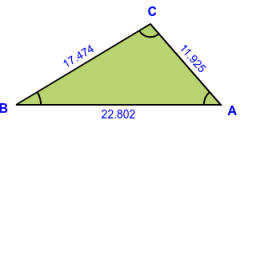# One angle

One angle is 10 degrees more than four times the measure of the other angle. Together they have a sum of 120 degrees. What is the measure of each angle?

α =  98 °
β =  22 °

### Step-by-step explanation:Did you find an error or inaccuracy? Feel free to write us. Thank you!

Tips for related online calculators
Do you have a linear equation or system of equations and looking for its solution? Or do you have a quadratic equation?